# MV-1015: Using Spline3D to Model Combustion Forces in an Engine

In this tutorial you will learn how to use Spline3D to model an input which depends on two independent variables.

For the tutorial, you will build a Single Cylinder Engine model similar to the one in Figure 1.
What are Spline 3Ds?
Spline3Ds are reference data plotted in three-dimensional coordinates which have two independent vectors or axis. These can be visualized as a number of 2D Splines (Curves) placed at regular intervals along a third axis. For instance, a bushing is generally characterized by a Force versus the Displacement curve. Let’s say, the Force versus displacement also varies with temperature. Effectively, there are two independent variables for the bushing force - Displacement and Temperature. Another example is the Engine Pressure (or Force) versus the Crank angle map (popularly known as P-Theta diagram). The P-theta map will vary at different engine speeds (or RPM). Such a scenario can be modeled using Spline3D.

In this exercise, engine mechanism is simulated where the combustion force that varies with regard crank angle and engine speed is modeled using Spline3D.

## Review the Model

In this step, you will load and review the model.

From the mbd_modeling\interactive\spline3d folder, copy the files SingleCylEngine.mdl and FTheta.csv to your <working directory>.
1. Start a new MotionView session.
2. Click the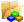(Open Model) icon to open the SingleCylEngine.mdl model file.
3. Review the model.
1. The model is a piston cylinder mechanism with a flywheel.
2. The model has two systems: System Cyl1 and System Flywheel.
4. Review the System Flywheel.
1. The Flywheel (fixed to Crank) is driven by a velocity based motion between markers. The markers refer to a curve (Crank_RPM) for inputs.
2. The curve Crank_RPM indicates the time history of crank speed during the simulation. The speed ramps up to 500RPM and then 1000, 1500, and 2000 RPM.
3. There are two solver variables: Crank_angle (deg) and Crank_RPM keep track of the angular rotation (in degrees) and velocity (in RPM) of the crank respectively.
4. Outputs are defined to measure the crank angle and RPM.
5. Review the System Cyl1.
1. The solver variables in System Flywheel are passed as attachments to this system and carry the variable names arg_Crank_angle_SolVar and arg_Crank_RPM_SolVar. You will use these to define the independent variables while defining the combustion force using Spline3D.
2. A Cumbustion_ref marker exists as a reference for a combustion force whose Z axis is aligned along the direction of travel of the piston.

In this step you will learn how to add a combustion force to the piston using Spline3D.

1. Add a Spline entity by dong one of the following:
• From the Project Browser, right-click on System Cyl1 and select Add > Reference Entity > Spline3D.
• In the Project Browser, Select System Cly1 and right-click the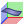(Spline3D) icon.
2. In the dialog, enter F_ThetaSpline as the Label and spl3d_F_ThetaSpline for the Variable.
3. Click OK.
This will display the Spline3D panel with the Properties tab active.
4. From the Type drop-down menu, select Value.
Note: The data for the spline can be defined using either the File or Value methods. For the File type, a reference to an external file in .csv format must be provided. In case of the Value type, the values can be imported from a .CSV file (using Import) or they can be entered in manually. In this tutorial, we will import the values from an external file.
5. Click the Import button to display the Import Values From File dialog.
6. From your <working directory>, browse to FTheta.csv and click OK.
7. In the Warning dialog that appears, click Yes.
The .csvfile that will be used as the source for Spline3D needs to be in the format shown in Figure 7.
• The first column must hold the X-axis values (shown in blue in Figure 7) which is the first independent variable.
• The top row holds the Z-axis values (shown in Figure 7) which is the second independent variable.
• The other columns must have the Y-axis values (shown in green in Figure 7) with each column belonging to the particular Z-axis values heading that column.
The same format is applicable when using the File input type.
Once imported, the values are populated in the panel.
8. In the Panel, you can review the values in the table by clicking the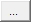(Expansion) button to open the Spline Values Table Data window.
Note: When manually keying in the values, context menus are available which allow you to Insert/Delete/Append row and column data. You can access these menus by right-clicking on any of the row or column headers. If the right-click is made on the last row/column, an Append option will also be available.
9. Close the Spline Values Table Data window.
10. Activate the Linear Extrapolation check box.
This will ensure that the values are extrapolated if the Solver starts looking for values beyond the range of the user provided data.
11. Click the Show Spline button to visualize the spline graphically.
You can view all three axes in an isometric view.
12. Click to Close the viewer.
The imported values are Combustion Force on Piston vs Theta (crank angle) diagrams at different speeds (as shown in Figure 14). The F-Theta profiles vary slightly at different engine or crank speeds. The same plot was visualized in the Spline3D Viewer by placing the four different plots along the Z-axis.

## Add a Force Using Spline3D

In this step you will add a force to represent the combustion in the cylinder. You will map it to the Spline3D added in Add a Spline3D Entity.

1. Add a force using one of the following methods:
• From the Project Browser, right-click on System Cyl1 and select Add > Force Entity > Force from the context menu.
• Select System Cyl1 in the Project Browser and then right-click on the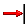(Force) icon on the Force Entity toolbar.
2. In the dialog, enter ThetaSpline Force as the Label and enter a Variable name. Click OK.
3. From the Force panel, in the Connectivity tab use the Force menu to select Action reaction.
4. Resolve the connections as shows in Figure 15.
1. Double click on the input collector (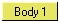and so on) and use the Model Tree.
2. Select the desired entity from the modeling window.
Note: The Body 2 reference to Ground Body is through an attachment to the System Cyl1 system.
5. From the Trans Properties tab, change the Fz type to Spline3D.
6. Double click on the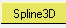(Spline3D collector).
7. From the Select a Spline3D dialog, expand System Cyl1 in the Model Tree and then select F_ThetaSpline.
8. Click OK.
9. In the Independent variable X field, enter the expression MOD({arg_Crank_angle_SolVar.VARVAL},720).
10. In the Independent variable Z field, enter {arg_Crank_RPM_SolVar.VARVAL}.
11. On the Model check toolbar, click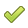(Check Model).
Note: The solver function MOD() used in Independent variable X refers to the solver variable Crank_angle (deg) in System Flywheel (via attachment arg_Crank_angle_SolVar to System Cyl1). This function calculates the remainder of the division of first argument value (value of the solver variable) by the second argument value (720); thereby resetting the value of Independent variable X every 720 degrees.
12. To save the model, from the menu bar click File > Save As > Model.

## Solve the Model

In this step, you will solve the model with MotionSolve.

1. On the General Actions toolbar, click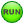(Run).
2. In the Run panel, enter an end time of 40 seconds and change the print interval to 0.001.
3. Click the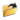(Open File) icon and assign a name and location for the MotionSolve XML file.
4. Click the Run button.
This will invoke MotionSolve and solve the model.
5. When the job is complete, Close the HyperWorks Solver View window and the Message Log.

## Post-Process the Results

In this step, you will post-process the MotionSolve results from your Spline3D model.

Before you begin, complete the step Solve the Model.
1. In the Run panel, click the Animate button.
This will load your results in a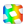HyperView window.
2. From the Animation toolbar, click the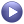(Start/Pause Animation) button to animate the model.
3. Visualize the forces on the Piston.
1. Click the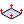(Vector) icon.
2. In the Vector panel, select the Piston graphics for the Assemblies collector.
You can also set all graphics to be transparent. From the Visualization toolbar, click WireFrame > Transparent Elements and Feature Lines.
4. From the Page Controls toolbar, click the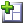(Add Page) icon.
5. In the Select Application drop-down menu, change the client to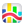HyperGraph 2D.
6. In the Page Controls toolbar, click the arrow next to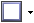(Page Window Layout) and click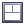(3 window layout).
7. From the Build Plots panel, click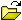to load the .plt file from the MotionSolve run.
8. Plot the Crank_angle (deg).
1. Click in the first window (top left) to make it the active window.
2. For Y Type, click User Defined.
3. For Y Request, click REQ/70000003 Crank_angle (deg).
4. For Y Component, click f3.
9. Plot the CombustionForce in the Z direction.
1. Click in the second window (top right) to make it the active window.
2. For Y Type, click Force.
3. For Y Request, click REQ/70000002 ThetaSpline Force on Piston (ForceOnPiston).
4. For Y Component, click Z.
10. Plot the Force vs Theta plots at different speeds as applied on the Piston.
This will demonstrate the usage of the Spline3D input used in the Add a Spline3D Entity step of this tutorial.
1. Click in the modeling window of the third window (bottom) to make it the active window.
2. On the Curves toolbar, click on the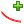(Define Curves) icon.
4. In the Curve field, rename the curve 500 RPM.
5. Change the source to Math.
6. In the x field, enter the expression p2w1c1.y[subrange(p2w1c1.x,6,7)]. In the y field, enter the expression p2w2c1.y[subrange(p2w2c1.x,6,7)]
7. Click Apply.
Note: p2w1c1 refers to the Curve 1 plotted on Page 2, Window 1. If for any reason the page, window, or curve numbering is different, suitable modifications should be made to the expression. The subrange function returns the indices of the vector within a specified range. For more information on the subrange function, please refer to the Templex and Math Reference Guide.
11. Add three more plots for 1000, 1500, and 2000 RPM. Use the expressions listed in Table 1.
Table 1.
Curve Name x expression y expression
1000 RPM p2w1c1.y[subrange(p2w1c1.x,16,17)] p2w1c1.y[subrange(p2w1c1.x,16,17)]
1500 RPM p2w1c1.y[subrange(p2w1c1.x,26,27)] p2w1c1.y[subrange(p2w1c1.x,26,27)]
2000 RPM p2w1c1.y[subrange(p2w1c1.x,36,37)] p2w1c1.y[subrange(p2w1c1.x,36,37)]
12. Assign different colors to these curves in one of the following ways:
• Click the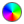(Curve Attributes) icon and select from the color panel under Line Attribute.
• In the Plot Browser, select each line and change the color in the Properties tab.
13. Compare the finished plots with the input data for the Spline3D plot in Add a Spline3D Entity.